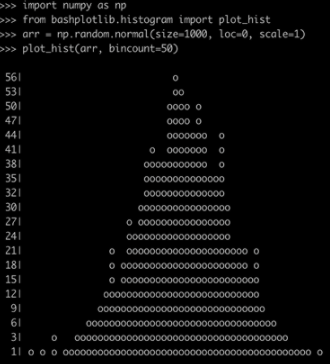• 写文章
• 提问

# 如何使用python3的Bashplotlib库绘制数据`pipinstall bashplotlib`

```importnumpy as np
from bashplotlib.histogram import plot_hist
arr = np.random.normal(size=1000, loc=0, scale=1)```

`plot_hist(arr, bincount=50)`